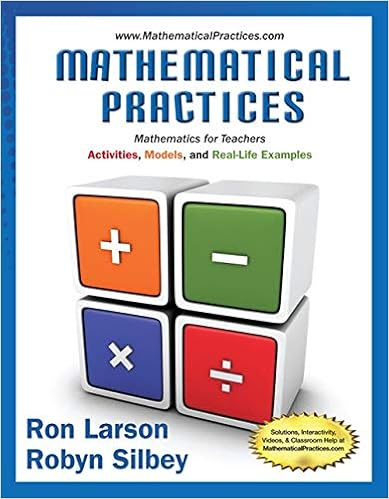S3 06-07 Maths Paper 2.doc - QUEENS COLLEGE YEARLY...

• Essay
• 13

This preview shows page 1 - 5 out of 13 pages.

We have textbook solutions for you!
The document you are viewing contains questions related to this textbook.The document you are viewing contains questions related to this textbook.
Chapter 4 / Exercise 3
Mathematical Practices, Mathematics for Teachers: Activities, Models, and Real-Life Examples
LarsonExpert Verified
QUEEN’S COLLEGE YEARLY EXAMINATION, 2006-2007 MATHEMATICS PAPER II Secondary: 3 Date: 13 TH June, 2007 Time: 10:30 – 11:30 (1 hour) INSTRUCTIONS 1. Answer all questions. You should mark all your answers on the MC Answer Sheet. 2. You should mark only ONE answer for each question. If you mark more than one answer, you will receive NO MARKS for that question. 3. Total Score of this paper II is 80 marks. 4. Calculator is allowed to be used. DO NOT TURN OVER THIS QUESTION BOOK UNTIL YOU ARE TOLD TO DO SO P.1
We have textbook solutions for you!
The document you are viewing contains questions related to this textbook.The document you are viewing contains questions related to this textbook.
Chapter 4 / Exercise 3
Mathematical Practices, Mathematics for Teachers: Activities, Models, and Real-Life Examples
LarsonExpert Verified
1. Simplify y x y x y x 2 3 2 2 2 5 ) 3 ( 9 .
2. Solve the exponential equation 4 2 x 4096.
3. The length of each side of a cube is increased by 10%. Find the percentage increase in the total surface area of the cube.
4. Mr. Chan deposits \$20 000 at a bank at an interest rate of 6% p.a. Find the simple interest that he will get after 1 year and 3 months. A. \$1 200 B. \$1 500 C. \$1 800 D. \$2 000
5. Factorize (5 x 2)(6 x 1) x 10 x + 4.
P.2
6. Factorize 3 3 250 16 e d .
P.3
A. AEH B. FEC C. BDE D. EC G 7. From the 2-D views provided below, find the corresponding solid. 8. In the figure, ABCDEFGH is a cube. Which of the following is not a right angle ?
9. What is the order of rotational symmetry of the solid shown below about the line l ?
•••